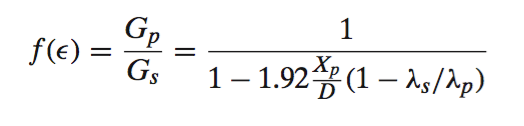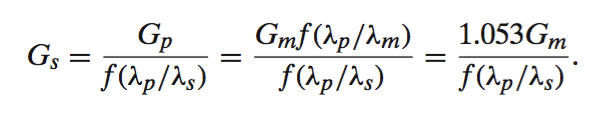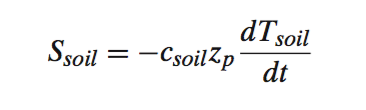Calculation of soil heat flux at the surface

### Preface

The following is lifted from "The energy balance experiment EBEX-2000: Part 1: overview and energy balance", Oncley, S.P., et al., 2007, Boundary-Layer Meteorology, 123:1--28, doi:10.1007/s10546-007-9161-1, updated with our procedure using current instrumentation.

### Introduction

The surface heat flux G(0) is determined from measurements by heat flux plates at a depth zp and the heat stored by the soil between the surface and zp. Thus

G(0) = Ssoil + G(zp).

#### Soil Heat Flux

G(zp) is measured using REBS heat flux plates (HFT) at a depth zp of 50 mm. The Philip (1961) correction for these plates is of the form (his Eq. 8):where Gp and Gs are the heat fluxes through the plate and through the soil, Xp = 3.93mm is the plate thickness, D = 38.56mm is the plate diameter, and λp = 1.22 W m1 K1 and λs are the thermal conductivities of the plate and soil, respec- tively. Our plates were calibrated by the manufacturer in a water/glass bead medium with thermal conductivity λm = 0.906 W m1 K1 and gave a measured value Gm based on this calibration coefficient. Thus, for our platesISFS now uses Hukseflux TP01 probes to measure soil thermal properties, including λs.  For earlier projects, we have sometimes estimated λfrom

λs = Qsoilλw + (1 Qsoild

where λw = 0.6 W m1 K1 is the thermal conductivity of water, Qsoil is the volumetric soil moisture fraction, and λmust be estimated for the local soil type.

#### Soil Heat Storage

Next, we need to calculate the heat storage in the soil above zpwhereand Tsoil is the soil temperature.  The TP01 now is used to measure csoil.   For earlier projects, we have simply used tables using estimates of the soil type.

Tsoil  is computed from the linear average of 4 sensors evenly spaced through the first 50mm of soil depth.  For some earlier projects, a single REBS linear soil temperature probe has been used positioned to average between 10 and 40 mm.

### Reference

Philip JR (1961) The theory of heat flux meters. J Geophys Res 66:571–579